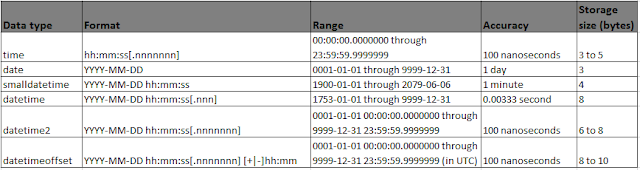SQL Server: Data Types - Part 2 - H Y R Tutorials
Date and Time Types
SQL server supports following date and time types

Date: Defines a date

Time [(fractional seconds precision)]: Define the time of day the time is without time zone awareness and is based on a 24-hour clock.

DateTime: Defines a date that is combined with the time of day with fractional seconds that is based on 24-hour clock.

SmallDateTime: Defines a date that is combined with the time of day the time is based on a 24-hour clock with seconds always (:00) and without fractional seconds.

DateTime2 [(Fractional seconds precision)]: Define a date that is combined with the time of day that is based on 24-hour clock datetime2 can be considered as an extension of the above DateTime type that has a larger date range.

Datetimeoffset [(Fractional seconds precision)]: Defines a date that is combined with the time of a day that has time zone awareness and is based on a 24-hour clock.Character Types
These are string data types of either fixed length or variable length

char[(n)]: Fixed length non-Unicode string data and defines the string length and must be a value from 1 to 8000 the storage size is n bytes.

varchar[(n/max)]: Variable length non-Unicode string data and defines the string length and can be a value from 1 to 8000 Max indicates that the maximum storage size is 2 ^ 31 - 1 byte (2 GB ).The storage size is the actual length of the data entered + 2 bytes.

text: same as varchar(Max).

nchar[(n)]: Fixed length Unicode string data. n defines the string length and must be a value from 1 to 4000 the storage size is 2 times of n bytes.

nvarchar[(n/max)]: Variable length Unicode string data. n defines the string length and can be a value from 1 to 4000 max indicates that the maximum storage size is 2^31 - 1 byte (2GB). the storage size in bytes is two times the actual length of data entered + 2 bytes.

ntext: Same as nvarchar(max).

In my next article, I will explain about remaining data types.